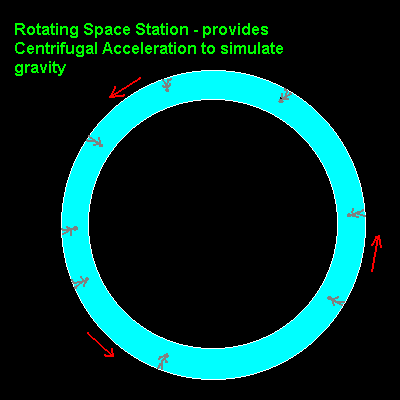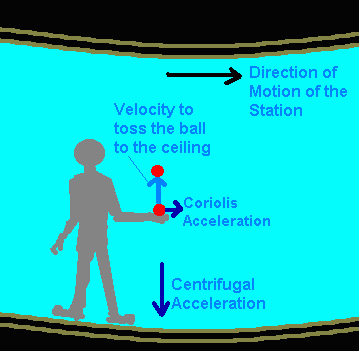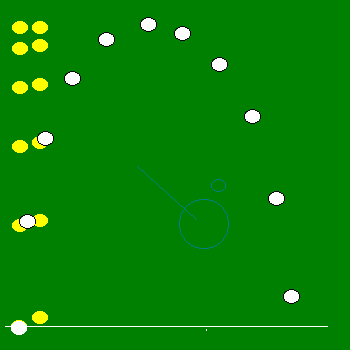## Rotating Space Station DynamicsAn Explanation of the Coriolis Force

by Larry Bogan
Nov 1998
 The Coriolis Force is not a real force in the sense that it is a result of any action by another body or field. It is a result describing motion on a rotating reference system. The most common rotating reference system is that of latitude and longitude of the Earth. The Earth rotates relatively slowly and the Coriolis Effect is not observable except in global size movements such as the circulation of the ocean waters or the movement of air in weather systems. It is not observable in the direction of rotation of water draining from a sink, contrary to popular literature. Very careful and specially designed experiments have shown the coriolis acceleration on a human scale but it is a very, very small effect easily obscured by small tilts and vibrations. An astronaut stationed on a rotating space station would easily see the effects of being in a rotating system. Although there is, at present, no rotating space station, the results can be predicted with relatively simple physics and geometry.

### Why Rotate a Space Station ?Two fictitious force occur in a rotating system. The more familiar of the two is the 'centrifugal force'. Everyone who has swung any heavy object around themselves has felt the centrifugal force. This 'force' can be used to simulate gravity in space where there is no solid surface to enable us feel the forces of gravity.

The centrifugal force is in a direction perpendicular to the rotation axis and radially outward. Its magnitude is equal to the square of the angular speed times the distance from the rotation axis. As a result the astronauts in the space station are able to walk around inside the space station as if the artifical gravity is pulling them outward away from the center of the toroidal (donut shaped) station.

For a space station 15 metres in radius (50 feet) the station must make one revolution about every 8 seconds in order that the astronauts feel 1 g of gravitational acceleration (ie. the his/her weight would be the same as that on Earth).

### Demonstation of Coriolis Acceleration in a Rotating Space StationOne very simple experiment that quickly illustrates the existance of the coriolis force is the tossing an object 'vertically' upward the 'ceiling'. On Earth the ball would fall back along the path upward and end back in your hand. In the space station this will not happen.

The coriolis force is always perpendicular the the direction of motion (velocity) of the ball and the axis of rotation of the station. For the ball going toward the center of the station the coriolis force will be in the direction of motion of the rim of the space station. Its magnitude is twice the product of the balls speed and the angular speed of the space station. When the ball reaches its maximum height (relative to the astronaut) its speed will be zero in the direction of the centre but the coriolis acceleration will have given the ball a horizontal velocity which results in a coriolis acceleration away from the centre. This causes the ball to 'fall' faster away from the center. As it falls, the coriolis acceleration is more back towards where it came from. As a result the ball follows an almost circular path. As the ball 'falls' in the artifically gravity due to the centrifugal force, its velocity will be away from the center of the space station and the coriolis force will be in the opposite direction . One might expect that the horizontal movement on the way up will be equal and opposite to that on the way down and the ball will end up at the astronauts hand. That is not the case.

### Mathematical SimulationI have calcuated the path of a ball tossed upward in a rotating station. The conditions are:
Distance from center at the start: 25 m
Centrifugal Acceleration at 25 m: 9.8 m/s2
Height of the toss: 1.5 m
The result is shown in the diagram to the right. The yellow ball shows the path of a 'normal' toss on the Earth. The white ball is shows the path of the ball on the space station. The ball positions are shown every 1/10th of a second.

Some of the calculated values are:
Angular velocity of the space station: 0.626 radians/s
Linear velocity of the station at 25 m: 15.65 m/s
Velocity of the ball at release: 5.68 m/s
Time of flight to maximum height: 0.512 seconds

Real time of flight is shown in the animation below.

To run the animation reload the page (from the menu or ctrl-r)Graphs showing the magnitudes of various quantities are listed below. In these graphs the quantities are separated in horizontal and vertical components as seen by the thrower of the ball.1. Displacements
2. Velocities
Note: the x-velocity increase and decreases - this is due to the existence of the coriolis acceleration. A ball thrown vertically on Earth would have x-velocity = 0 all the time. The y-velocity is very similar to that of a normal ball toss.
3. Accelerations
Note the x-acceleration is not quite the coriolis acceleration because it is not only in the horizontal direction. The y-acceleration is not constant for two reasons (1) the centrifugal acceleration depends on distance from the center of rotations and (2) the coriolis acceleration has a component in this direction. The x-acceleration which is entire due to the coriolis acceleration show how it changes sign as the ball reaches its maximum height half way through the flight.
The diagram to the right illustrates the directions and relative magnitudes of the centrifugal and coriolis acceleration during the flight of the ball.

### The Real Path of the Ball and HandThe easiest way to see what really is happening during the flight of the ball, is to observe the whole event from outside the rotating space station. From this non-rotating, point-of-view, the ball travels a straight path and the hand that threw the ball follows the curve of the rotation. This is shown in the diagram to the right. The ball is traveling a shorter path and at a faster speed, hence it will reach the 25 m radius before the hand gets there. This appears to the thrower that the ball has move sideways due to the 'strange' coriolis force.

### Velocity Dependent Weight of the Astronauts

 As we saw with the ball toss, at the top of the path, when the velocity of the ball was horizontal, the coriolis acceleration was downward (or outward relative to the rotating space station). If an astronaut runs in the direction of rotation of the space station, he will feel this acceleration as an added force on the floor, ie, his/her weight will effectively increase. If the astronaut runs in the opposite direction of the rotation, their weight will decrease! Some numbers will make this more real. In the same space station as described above, if you were to run at 1 m/s you would change your weight by 13%. Coriolis Acceleration = 2(0.626 rad/s)(1 m/s) = 1.26 m/s2 Relative to the centifugal force = 1.26 m/s2/9.8 m/s2 = 0.127 Of course what is happening as seen from outside the space station is that as the astronaut runs in the same direction as the space station, their speed in a circle increases and the force needed to move them in that circle must increase - hence the increase in 'weight'. When the astronaut runs opposite to the rotation of the station, their circular speed decreases and less radial acceleration is needed to move them in the cirele, hence a lower for on their feet and less 'weight'.

Copywrite Novemever 1998, L.Bogan